# GRE Quantitative Reasoning - Exponents: Help and Review Chapter Exam

Exam Instructions:

Choose your answers to the questions and click 'Next' to see the next set of questions. You can skip questions if you would like and come back to them later with the yellow "Go To First Skipped Question" button. When you have completed the practice exam, a green submit button will appear. Click it to see your results. Good luck!

### Page 1

#### Question 1 1. Which of the following is equivalent to the expression below?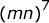#### Question 2 2. Simplify the following expression.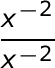#### Question 3 3. Using positive exponents, simplify the expression.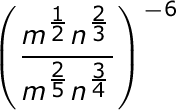#### Question 4 4. Which of the following would be true regarding the following exponential expression?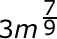#### Question 5 5. Simplify the following expression using positive exponents.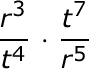### Page 2

#### Question 6 6. Simplify using ONLY positive exponents.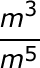#### Question 7 7. Simplify using only positive exponents.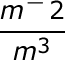#### Question 9 9. Solve, to 2 decimal places, the exponential equation below for x: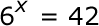#### Question 10 10. Simplify this expression using positive exponents.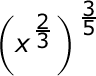### Page 3

#### Question 11 11. Which of the following is equivalent to the expression below?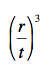#### Question 12 12. Which of the following radical expressions is equal to this exponential expression?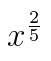#### Question 13 13. Simplify the expression below using positive exponents.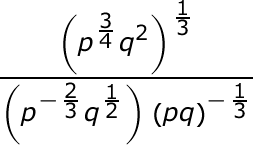#### Question 14 14. Simplify the expression below.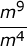### Page 4

#### Question 16 16. Simplify using ONLY positive exponents.#### Question 17 17. Which of the following is equivalent to the following expression?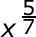#### Question 18 18. Solve the exponential equation below for x: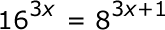#### Question 19 19. Which of the following is equivalent to the expression below?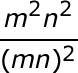#### Question 20 20. Simplify the expression below using only positive exponents.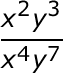### Page 5

#### Question 21 21. Simplify the expression below using positive exponents only.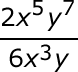#### Question 23 23. Simplify the expression using positive exponents.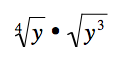#### Question 24 24. Solve the exponential equation below for x: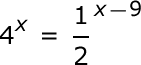#### Question 25 25. Which of the following exponential expressions is equal to this radical expression?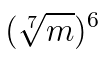### Page 6

#### Question 26 26. Simplify the following expression.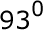#### Question 29 29. Which of the following is equivalent to the expression below?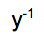#### GRE Quantitative Reasoning - Exponents: Help and Review Chapter Exam Instructions

Choose your answers to the questions and click 'Next' to see the next set of questions. You can skip questions if you would like and come back to them later with the yellow "Go To First Skipped Question" button. When you have completed the practice exam, a green submit button will appear. Click it to see your results. Good luck!

Support